# CBSE Class 9 Maths Surface Areas and Volumes MCQs

## Surface Areas and Volumes Class 9 MCQ

Class 9 Mathematics students should refer to the following multiple-choice questions with answers for Surface Areas and Volumes in standard 9. These MCQ questions with answers for Grade 9 Mathematics will come in exams and help you to score good marks

### Class 9 Surface Areas and Volumes MCQ Questions with Answers

Question: Each side of a cube is increased by 50%. Then the surface area of the cube increases by
a) 50%
b) 100%
c) 150%
d) 125%

Question: Instead of walking along two adjacent sides of a rectangular field, a boy took a short cut along the diagonal and saved a distance equal to half the longer side. Then the ratio of the shorter side to the longer side is
a) 3/4
b) 2/3
c) 1/2
d) 1/4

Question: The number of surfaces in right circular cylinder is
a) 4
b) 2
c) 1
d) 3

Question: A covered wooden box has the inner measures as 115 cm, 75 cm and 35 cm. Find the volume of the wood.
a) 82,125 cu cm
b) 85,000 cu cm
c) 84,000 cu cm
d) 80,000 cu cm

Question: The edge of a cube is 20 cm. How many small cubes of 5 cm edge can be formed from this cube?
a) 64
b) 4
c) 32
d) 100

Question: A metallic right circular cone of height 9 cm and base radius 7 cm is melted into a cuboid whose two sides are 11 cm and 6 cm. What is the third side of the cuboid?
a) 7 cm
b) 5 cm
c) 6 cm
d) 10 cm

Question: The slant height of a cone is increased by P%. If radius remains same, the curved surface area is increased by
a) P%
b) 2P%
c) P2%
d) None of these

Question: The dimensions of a hall are 40 m, 25 m and 20 m. if each person requires 200 cubic metre, then the number of persons who can be accommodated in the hall are
a) 100
b) 150
c) 120
d) 140

Question: A hollow spherical ball whose inner radius is 4 cm is full of water. Half of the water is transferred to a conical cup and it completely filled the cup. If the height of the cup is 2 cm, then the radius of the base of cone, in cm is
a) 8
b) 4
c) 16
d) 12

Question: The radius of the cylinder whose lateral surface area is 704 cm2 and height 8 cm is
a) 14 cm
b) 4 cm
c) 6 cm
d) 8 cm

Question: The radius of cylinder is doubled but its lateral surface area is unchanged. Then its height must be
a) halved
b) constant
c) doubled
d) tribled

Question: The ratio of the volume and surface area of a sphere of unit radius
a) 1 : 3
b) 4 : 3
c) 3 : 4
d) 3 : 1

Question: A cylindrical rod whose height is 8 times of its radius is melted and recast into spherical balls of same radius. The number of balls will be
a) 6
b) 4
c) 3
d) 8

Question: A sphere and a cube are of the same height. The ratio of their volume is
a) 11 : 21
b) 21 : 11
c) 3 : 4
d) 4 : 3

Question. The surface area of a cuboid is
(a) 2(lb + bh + lh)
(b) 3(lb + bh + lh)
(c) 2(lb – bh – lh)
(d) 3(lb – bh – lh)

Question. The surface area of a cube if edge ‘a’ is
(a) 7a2
(b) 6a2
(c) 5a3
(d) 5a2

Question. The length, breadth and height of a room is 5m, 4m and 3m. The cost of white washing its four walls at the rate of Rs. 7.50 per m2 is
(a) Rs. 110
(b) Rs. 109
(c) Rs. 220
(d) Rs. 105

Question. The perimeter of floor of rectangular hall is 250m. The cost of the white washing its four walls is Rs. 15000. The height of the room is
(a) 5m
(b) 4m
(c) 6m
(d) 8m

Question. The breadth of a room is twice its height and is half of its length. The volume of room is 512dm3. Its dimensions are
(a) 16 dm, 8 dm, 4 dm
(b) 12 dm, 8 dm, 2 dm
(c) 8 dm, 4 dm, 2 dm
(d) 10 dm, 15 dm, 20 dm

Question. The area of three adjacent faces of a cube is x, y and z. Its volume V is
(a) V = xyz
(b) V3 = xyz
(c) V2 = xyz
(d) none of these

Question. Two cubes each of edge 12 cm are joined. The surface area of new cuboid is
(a) 140 cm2
(b) 1440 cm2
(c) 144 cm2
(d) 72 cm2

Question. The curved surface area of cylinder of height ‘h’ and base radius ‘r’ is
(a) 2∏rh
(b) ∏rh
(c) 1/2∏rh
(d) none of these

Question. The total surface area of cylinder of base radius ‘r’ and height ‘h’ is
(a) 2π(r + h)
(b) 2πr(r + h)
(c) 3πr(r + h)
(d) 4πr(r + h)

Question. The curved surface area of a cylinder of height 14 cm is 88 cm2. The diameter of its circular base is
(a) 5cm
(b) 4cm
(c) 3cm
(d) 2cm

Question. It is required to make a closed cylindrical tank of height 1 m and base diameter 140cm from a metal sheet. How many square meters a sheet are required for the same?
(a) 6.45m2
(b) 6.48m2
(c) 7.48m2
(d) 5.48m2.

Question. A metal pipe is 77 cm long. Inner diameter of cross section is 4 cm and outer diameter is 4.4 cm. Its inner curved surface area is:
(a) 864 cm2
(b) 968 cm2
(c) 768 cm2
(d) none of these

Question: Vertical and horizontal cross-sections of a right circular cylinder are always respectively

• a) rectangle, circle
• b) rectangle, ellipse
• c) square, circle
• d) rectangle, square

• a) 3
• b) 2
• c) 4
• d) 5

• a) 8 cm
• b) 6 cm
• c) 4 cm
• d) 9 cm

• a) 1 : 2
• b) 1 : 4
• c) 1 : 1
• d) 1 : 3

• a) 96 m
• b) 104 m
• c) 208 m
• d) 406 m

#### Question: A metal sheet 27 cm long, 8 cm broad and 1 cm thick is melted into a cube. The difference between surface areas of two solids is

• a) 286 cm2
• b) 280 cm2
• c) 284 cm2
• d) None of these

• a) 6 cm
• b) 9 cm
• c) 12 cm
• d) 10 cm

#### Question: If length, breadth and height of rectangular parallelopiped are in ratio 6 : 5 : 4 and total surface area\ is 33,300 m2, then length breadth and height of parallelopiped are in (m)

• a) 90, 75, 60
• b) 90, 75 , 70
• c) 85, 75, 60
• d) 80, 70, 60

• a) 14 cm
• b) 4 cm
• c) 6 cm
• d) 8 cm

#### Question: A covered wooden box has the inner measures as 115 cm, 75 cm and 35 cm and the thickness of wood is 2.5 cm. The volume of the wood, is

• a) 82,125 cu cm
• b) 85,000 cu cm
• c) 80,000 cu cm
• d) 84,000 cu cm

• a) 120
• b) 96
• c) 108
• d) 100

• a) 1 : 9
• b) 1 : 3
• c) 1 : 6
• d) 1 : 18

• a) 24
• b) 60
• c) 48
• d) 324

#### Question: A rectangle field has its length and breadth in the ratio 5 : 3. Its area is 3.75 hectares. The cost of fencing it at Rs. 5 per meter is

• a) Rs. 4000
• b) Rs. 500
• c) Rs. 1000
• d) None of these

Question: The diameter of a roller is 84 cm and its length is 120 cm. It takes 500 complete revolutions to move once over to level a playground. The area of the playground in m2 is:
(a) 1584
(b) 1284
(c) 1384
(d) 1184

Question: A cylindrical pillar is 50 cm in diameter and 3.5 m in height. The cost of painting its curved surface at the rate of Rs. 12.50 per m2 is:
(a) Rs. 68.75
(b) Rs. 58.75
(c) Rs. 48.75
(d) Rs. 38.75

Question: The inner diameter of circular well is 3.5m. It is 10m deep. Its inner curved surface area in m2 is:
(a) 120
(b) 110
(c) 130
(d) 140

Question: In a hot water heating system there is a cylindrical pipe of length 28 m and diameter 5 cm. The total radiating surface area in the system in m2 is:
(a) 6.6
(b) 5.5
(c) 4.4
(d) 3.4

Question: The curved surface area of a right circular cone of slant height 10 cm and base radius 7 cm is
(a) 120 cm2
(b) 220 cm2
(c) 240 cm2
(d) 140 cm2

Question: The height of a cone is 16 cm and base radius is 12 cm. Its slant height is
(a) 10 cm
(b) 15 cm
(c) 20 cm
(d) 8 cm

Question: The curved surface area of a right circular cone of height 16 cm and base radius 12 cm is
(a) 753.6 cm2
(b) 1205.76 cm2
(c) 863.8 cm2
(d) 907.6 cm2

Question: The curved surface area of a right circular cone of slant height 10 cm and base radius 10.5 cm is
(a) 185 cm2
(b) 160 cm2
(c) 165 cm2
(d) 195 cm2

Question: The slant height of a cone is 26 cm and base diameter is 20 cm. Its height is
(a) 24 cm
(b) 25 cm
(c) 23 cm
(d) 35 cm

Question: The curved surface area of a cone is 308 cm2 and its slant height is 14 cm. The radius of its baseis
(a) 8 cm
(b) 7 cm
(c) 9 cm
(d) 12 cm

Question:  A conical tent is 10 m high and the radius of its base is 24 m. The slant height of tent is
(a) 26 m
(b) 28 m
(c) 25 m
(d) 27 m

Question: The slant height and base diameter of a conical tomb are 25 m and 14 m respectively. The cost of white washing its curved surface at the rate of Rs. 210 per 100 m2 is
(a) Rs. 1233
(b) Rs. 1155
(c) Rs. 1388
(d) Rs. 1432

Question. A joker’s cap is in the form of cone of base radius 7 cm and height 24 cm. The area of sheet to make 10 such caps is
(a) 5500 cm2
(b) 6500 cm2
(c) 8500 cm2
(d) 3500 cm2

Question. The curved surface area of a hemisphere of radius ‘r’ is
(a) 2∏r2
(b) 4∏r2
(c) 3∏r2
(d) 5∏r2

Question. The total surface area of a hemisphere of radius ‘r’ is
(a) 2∏r2
(b) 4∏r2
(c) 3∏r2
(d) 5∏r2

Question. The curved surface area of a sphere of radius 7 cm is:
(a) 516 cm2
(b) 616 cm2
(c) 716 cm2
(d) 880 cm2

Question. The curved surface area of a hemisphere of radius 21 cm is:
(a) 2772 cm2
(b) 2564 cm2
(c) 3772 cm2
(d) 4772 cm2

Question. The curved surface area of a sphere of radius 14 cm is:
(a) 2464 cm2
(b) 2428 cm2
(c) 2464 cm2
(d) none of these.

Question. The curved surface area of a sphere of diameter 14 cm is:
(a) 516 cm2
(b) 616 cm2
(c) 716 cm2
(d) 880 cm2

Question. Total surface area of hemisphere of radius 10 cm is
(a) 942 cm2
(b) 940 cm2
(c) 842 cm2
(d) 840 cm2

Question. The radius of a spherical balloon increases from 7 cm to 14 cm s air is being pumped into it. The ratio of surface area of the balloon in the two cases is:
(a) 4 : 1
(b) 1 : 4
(c) 3 : 1
(d) 1 : 3

Question. A matchbox measures 4 cm x 2.5 cm x 1.5 cm. The volume of packet containing 12 such boxes is:
(a) 160 cm3
(b) 180 cm3
(c) 160 cm2
(d) 180 cm2

Question. A cuboidal water tank is 6 m long, 5 m wide and 4.5 m deep. How many litre of water can it hold?
(a) 1350 liters
(b) 13500 liters
(c) 135000 liters
(d) 135 liters

Question. A cuboidal vessel is 10 m long and 8 m wide. How high must it be made to hold 380 cubic metres of a liquid?
(a) 4.75 m
(b) 7.85 m
(c) 4.75 cm
(d) none of these

Question. The capacity of a cuboidal tank is 50000 litres. The length and depth are respectively 2.5 m and 10 m. Its breadth is
(a) 4 m
(b) 3 m
(c) 2 m
(d) 5 m

Question. A godown measures 40 m × 25 m × 10 m. Find the maximum number of wooden crates each measuring 1.5 m × 1.25 m × 0.5 m that can be stored in the godown.
(a) 18000
(b) 16000
(c) 15000
(d) 14000

Question. A river 3 m deep and 40 m wide is flowing at the rate of 2 km per hour. How much water will fall into the sea in a minute?
(a) 4000 m3
(b) 40 m3
(c) 400 m3
(d) 40000 m3

Question. The circumference of the base of a cylindrical vessel is 132 cm and its height is 25 cm. How many litres of water can it hold?
(a) 33.75 litre
(b) 34.65 litre
(c) 35.75 litre
(d) 38.75 litre

Question. If the lateral surface of a cylinder is 94.2 cm2 and its height is 5 cm, then find radius of its base
(a) 5cm
(b) 4cm
(c) 3cm
(d) 6cm

Question. It costs Rs 2200 to paint the inner curved surface of a cylindrical vessel 10 m deep. If the cost of painting is at the rate of Rs 20 per m2, find radius of the base,
(a) 1.75 m
(b) 1.85 m
(c) 1.95 m
(d) 1.65 m

Question. The height and the slant height of a cone are 21 cm and 28 cm respectively. Find the volume of the cone.
(a) 5546 cm3
(b) 7546 cm3
(c) 5564 m3
(d) 8546 cm3

Question. Find the volume of the right circular cone with radius 6 cm, height 7 cm
(a) 254 cm3
(b) 264 cm3
(c) 274 cm2
(d) 284 cm3

Question. The radius and height of a conical vessel are 7 cm and 25 cm respectively. Its capacity in litres is
(a) 1.232 litre
(b) 1.5 litre
(c) 1.35 litre
(d) 1.6 litre

Question. The height of a cone is 15 cm. If its volume is 1570 cm3, find the radius of the base.
(a) 12 cm
(b) 10 cm
(c) 15 cm
(d) 18 cm

Question. If the volume of a right circular cone of height 9 cm is 48π cm3, find the diameter of its base.
(a) 12 cm
(b) 10 cm
(c) 6 cm
(d) 8 cm

Question. A conical pit of top diameter 3.5 m is 12 m deep. What is its capacity in kilolitres?
(a) 38.5 kl
(b) 48.5 kl
(c) 39.5 kl
(d) 47.5 kl

Question. Find the capacity in litres of a conical vessel with radius 7 cm, slant height 25 cm
(a) 1.232 litre
(b) 1.5 litre
(c) 1.35 litre
(d) none of these

Question. The diameter of the moon is approximately one-fourth of the diameter of the earth. What fraction of the volume of the earth is the volume of the moon?
(a) 1/64
(b) 1/ 32
(c) 1/16
(d) 1/48

Question. The dimensions of a cuboid are 50 cm x 40 cm x 10 cm. Its volume in litres is:
(a) 10 litres
(b) 12 litres
(c) 20 litres
(d) 25 litres

Question. The volume of a cuboidal tank is 250 m3. If its base area is 50 m2 then depth of the tank is
(a) 5 m
(b) 200 m
(c) 300 m
(d) 12500 m

Question. The length, breadth and height of a cuboidal solid is 4 cm, 3 cm and 2 cm respectively. Its volume is
(a) (4 + 3 +2) cm3
(b) 2(4 + 3 +2) cm3
(c) (4 x 3 x 2) cm3
(d) 2(4 + 3) x 2 cm3

Question. The volume of a cuboidal solid of length 8 m and breadth 5 m is 200 m3. Find its height.
(a) 5 m
(b) 6 m
(c) 15 m
(d) 18 m

Question. The curved surface area of a sphere is 616 cm2. Its radius is
(a) 7 cm
(b) 5 cm
(c) 6 cm
(d) 8 cm

Question. If radius of a sphere is 2d/3 then its volume is
(a) 32/81πd3
(b) 23/4πd3
(c) 32/3πd3
(d) 34/3πd3

Question. The capacity of a cylindrical tank is 6160 cm3. Its base diameter is 28 m. The depth of this tank is
(a) 5 m
(b) 10 m
(c) 15 m
(d) 8 m

Question. The volume of a cylinder of radius r and length h is:
(a) 2πrh
(b) 4/3πr2h
(c) πr2h
(d) 2πr2h

Question. Base radius of two cylinder are in the ratio 2 : 3 and their heights are in the ratio 5 : 3. The ratio of their volumes is
(a) 27 : 20
(b) 25 : 24
(c) 20 : 27
(d) 15 : 20

Question. If base radius and height of a cylinder are increased by 100% then its volume increased by:
(a) 30%
(b) 40%
(c) 42%
(d) 33.1%

Question. The diameter of a sphere is 14 m. The volume of this sphere is
(a) 1437 1/3 m3
(b) 1357 1/3 m3
(c) 1437 2/3 m3
(d) 1337 2/3 m3

Question. The volume of a sphere is 524 cm3. The diameter of sphere is
(a) 5cm
(b) 4cm
(c) 3cm
(d) 7cm

Question. The total surface area of a cylinder is 40π cm2. If height is 5.5 cm then its base radius is
(a) 5cm
(b) 2.5cm
(c) 1.5cm
(d) 10cm

Question. The area of circular base of a right circular cone is 78.5 cm2. If its height is 12 cm then its volume is
(a) 31.4 cm3
(b) 3.14 cm3
(c) 314 cm3
(d) none of these

Question. The base radius of a cone is 11.3 cm and curved surface area is 355 cm2. Its height is (Take π = 355/113)
(a) 5 cm
(b) 10 cm
(c) 11 cm
(d) 9 cm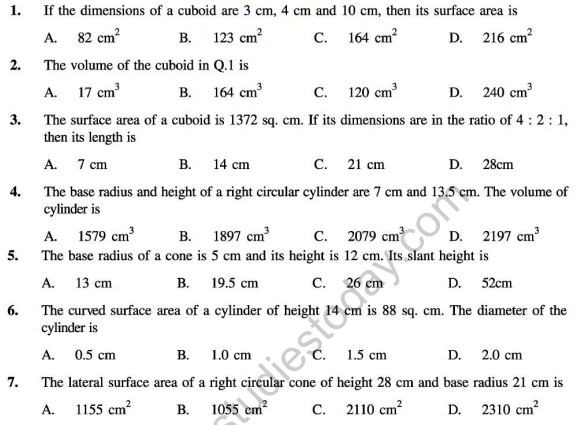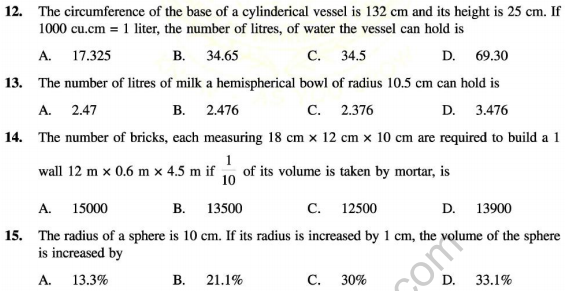1. The space occupied by a solid body is called its volume. The units of volume are cubic centimetre (cm3) or cubic metres (m3) etc.

2. Cuboid : A solid bounded by six rectangular plane faces is called a cuboid.

3. Cube : A cuboid whose length, breadth and height are all equal is called a cube.

4. Cuboid : For a cuboid of length = l units, breadth = b units and height = h units.
(i) Volume of cuboid = (l × b × h) cubic units
(ii) Diagonal of the cuboid = √l2 + b2 + h2 units
(iii) Total surface area of the cuboid = 2(lb + bh + lh) sq units
(iv) Lateral surface area of the cuboid = [2(l + b) × h] sq units
(v) Area of 4 walls of a room = [2(l + b) × h] sq units

5. Cube : For a cube of edge = a units
(i) Volume of the cube = a3 cubic units
(ii) Diagonal of the cube = a √3 units
(iii) Total surface area of the cube = (6a2) sq units
(iv) Lateral surface are of the cube = (4a2) sq units
Relation between units
Length units Volume units
1 cm = 10 mm 1 cm2 = 1000 mm3
100 cm = 1 m 1 m3 = 1000000 cm3
1 litre = 1000 cm3
1 kilolitre = 1000 litres = 1 m3

6. Cylinder : A solid having a curved surface as a lateral surface and a uniform circular cross-section is known as a cylinder

b If the axis of the cylinder is perpendicular to each cross-section then the cylinder is called a right circular cylinder7. Volume of a cylinder : For a cylinder whose height is a h units and the radius of whose base is r units, Volume of cylinder = (πr2h) cubic units = (base area) × height

8. Surface area of cylinder : For a cylinder of height h and radius r :
(a) Area of curved surface = (2 πrh) sq units
(b) Total surface area = (2πrh + 2π r2) sq units

9. Cone : It is a solid having a plane circular end as the base and whose lateral surface is a curved surface tapering into a point, called its vertex.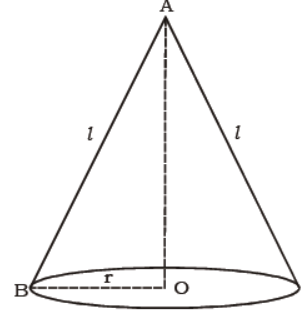Note : For a right circular cone, we have AO is perpendicular to OB, i.e., ΔAOB is right angled at O. Hence, l2 = r2 + h2

10. Volume of a cone : For a cone of radius r units and height h units, volume of cone = (1/3 πr2h) cubic units.

11. Area of curved surface : For a cone of radius r, height h and slant height l,
(a) Area of curved surface = prl sq units
(b) Total surface area = (πrl + πr2) sq units

12. (a) Sphere : The set of all points in space equidistant from a fixed point is called a sphere.
(b) Hemisphere : A plane through the centre of a sphere cuts it into two equal parts. Each part is called a hemisphere.
(c) Spherical shell : The difference of two solid concentric spheres is called a spherical shell.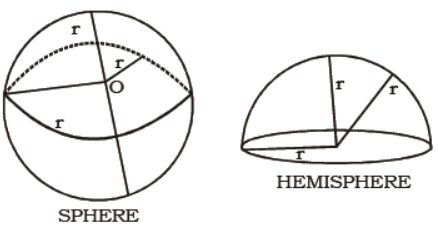13. For a sphere of radius r units :
(a) Volume of sphere = (1/3πr3) cubic units
(b) Area of curved surface of the sphere = (4πr2) cubic units
(c) Volume of the hemisphere = (1/3πr3)cubic units
(d) Curved surface of the hemisphere = (2πr2) sq units
(e) Total surface area of the hemisphere = 2πr2 + πr2 = (3πr2) sq units

Question. A cooking pot has a spherical bottom, while the upper part is a truncated cone. Its vertical crosssection is shown in the figure. If the volume of food increases by 15% during cooking, the maximum initial volume of food that can be cooked without spilling is (in cc)(A) 14450 π/3
(B) 19550  π/3
(C) 340000/69π
(D) 20000/3 π

Question. Each side of a cube is increased by 50%. Then the surface area of the cube increases by
(A) 50%
(B) 100%
(C) 125%
(D) 150%

Question. Three cylinders each of height 16 cm and radius of base 4 cm are placed on a plane so that each cylinder touches the other two. Then the volume of region enclosed between the three cylinders in cm3 is
(A) 98(4 √3 - π)
(B) 98(2 √3 - π)
(C) 98( √3 - π)
(D) 128(2 √3 - π)

Question. Instead of walking along two adjacent sides of a rectangular field, a boy took a short cut along the diagonal and saved a distance equal to half the longer side. Then the ratio of the shorter side to the longer side is
(A) 1/2
(B) 2/3
(C) 1/4
(D) 3/4

Question. The number of surfaces in right circular cylinder is
(A) 1
(B) 2
(C) 3
(D) 4

Question.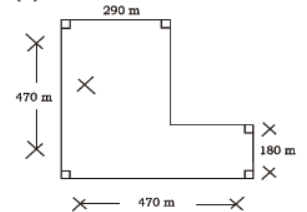Fields X and Y are to be enclosed with a fencing at the cost of Rs 40 per meter. If the cost on field X is denoted by Cx and that on field Y is denoted by Cy we have
(A) Cx = Cy
(B) Cx < Cy
(C) Cx > Cy
(D) cannot be determined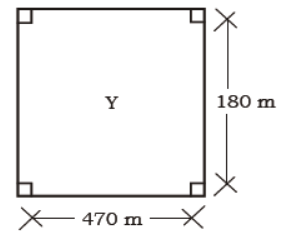Question. A covered wooden box has the inner measures as 115 cm, 75 cm and 35 cm. Find the volume of the wood.
(A) 80,000 cu cm
(B) 82,125 cu cm
(C) 84,000 cu cm
(D) 85,000 cu cm

Question. The edge of a cube is 20 cm. How many small cubes of 5 cm edge can be formed from this cube?
(A) 4
(B) 32
(C) 64
(D) 100

Question. Two cylinders of same volume have their heights in the ratio 1 : 3. Find the ratio of their radii.
(A) √3 :1
(B) √2 :1
(C) √5 : 2
(D) 2: √5

Question. A metallic right circular cone of height 9 cm and base radius 7 cm is melted into a cuboid whose two sides are 11 cm and 6 cm. What is the third side of the cuboid?
(A) 5 cm
(B) 6 cm
(C) 7 cm
(D) 10 cm

Question. The slant height of a cone is increased by P%. If radius remains same, the curved surface area is increased by
(A) P%
(B) P2%
(C) 2P%
(D) None

Question. The volumes of two spheres are in the ration 64 : 27. Find the difference of their surface areas, if the sum of their radii is 7 units
(A) 28π sq units
(B) 88 sq units
(C) 88π sq units
(D) 4π sq units

Question. In the figure below, LMNO and GHJK are rectangles where GH = 1/2 LM and HJ = 1/2 MN. What fraction of the region is bounded by LMNO that is not shaded?
(A) 1/4
(B) 1/3
(C) 1/2
(D) 3/4

Question. In the figure below, RSTV is a square inscribed in a circle with centre O and radius r. The total are of shaded region is
(A) r2(π – 2)
(B) 2r(2 – π)
(C) π(r2 – 2)
(D) π r2 – 8r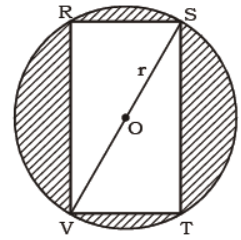Question. A right circular cone of diameter K cm and height 12 cm rests on the base of a right circular cylinder of radius K cm (their bases lie in the same plane, as shown in figure). The cylinder is filled with water to a height of 12 cm. If the cone is then removed, the height to which water will fall is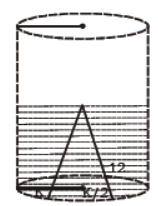(A) 11 cm
(B) 10 cm
(C) 8 cm
(D) cannot be determined from given data

Question. The ratio of radii of two cylinders is 1 : √3 and heights are in the ratio 2 : 3. The ratio of their volumes is
(A) 1 : 9
(B) 2 : 9
(C) 4 : 9
(D) 5 : 9

Question. The dimensions of a hall are 40 m, 25 m and 20 m. if each person requires 200 cubic metre, then the number of persons who can be accommodated in the hall are
(A) 120
(B) 150
(C) 140
(D) 100

Question. Correct the perimeter of the figure given below to one decimal place.(A) 56.0 m
(B) 56.6 m
(C) 57.2 m
(D) 57.9 m

Question. A hollow spherical ball whose inner radius is 4 cm is full of water. Half of the water is transferred to a conical cup and it completely filled the cup. If the height of the cup is 2 cm, then the radius of the base of cone, in cm is
(A) 4
(B) 8π
(C) 8
(D) 16

Question. The largest volume of cube that can be inclosed in a sphere of diameter 2 cm is (in cm3)
(A) 1
(B) 2 √2
(C) π
(D) 8/3√3

Question. The radius of the cylinder whose lateral surface area is 704 cm2 and height 8 cm is
(A) 6 cm
(B) 4 cm
(C) 8 cm
(D) 14 cm

Question. The radius of a sphere is increased by P%. Its surface area increases by
(A) P%
(B) P2%
(C) 8(2P+P2/100)%
(D) P2/P %

Question. The radius of cylinder is doubled but its lateral surface area is unchanged. Then its height must be
(A) doubled
(B) halved
(C) tribled
(D) constant

Question. The height and radius of a cone are 3 cm and 4 cm respectively. Its curved surface area must be is
(A) 62x 6/7 sq cm
(B) 57x 3/4 sq cm
(C) 6 cm2
(D) 12 cm2

Question. The ratio of the volume and surface area of a sphere of unit radius
(A) 4 : 3
(B) 3 : 4
(C) 1 : 3
(D) 3 : 1

Question. A cylindrical rod whose height is 8 times of its radius is melted and recast into spherical balls of same radius. The number of balls will be
(A) 4
(B) 3
(C) 6
(D) 8

Question. Two identical right circular cones each of height 2 cm are placed as shown in diagram (each is vertical, apex downward). At the start, the upper cone is full of water and lower cone is empty.
Then water drips down through a hole in the apex of upper cone into the lower cone. The height of water in the lower cone at the moment when height of water in upper cone is 1 cm is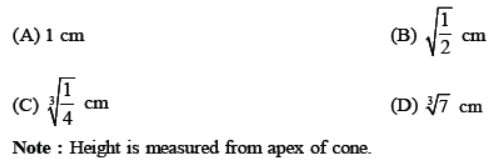Question. A sphere and a cube are of the same height. The ratio of their volume is
(A) 3 : 4
(B) 21 : 11
(C) 4 : 3
(D) 11 : 21

Question. The largest sphere is cut off from a cube of side 5 cm. The volume of the sphere will be
(A) 27 π cm3
(B) 30 π cm3
(C) 108 π cm2
(D) 125 π/6 cm3

Question. Vertical and horizontal cross-sections of a right circular cylinder are always respectively
(A) rectangle, square
(B) rectangle, circle
(C) square, circle
(D) rectangle, ellipse

Question. A right cylindrical vessel is full with water. How many right cones having same diameter and height as of right cylinder will be needed to store that water?
(A) 2
(B) 4
(C) 3
(D) 5

Question. Two cylinders of same volume have their heights in the ration 1 : 3. Then the ratio of their radii is
(A) √3 :1
(B) √2 :1
(C) √5 : 2
(D) 2: v5

Question. ABC is a right angled triangle with ∠ABC = 90º . AH is drawn perpendicular to BC where H lies on BC. If AB = 60 cm and AC = 80 cm, then BH =(A) 36 cm
(B) 32 cm
(C) 24 cm
(D) 30 cm

Question. The area of a trapezium is 28 cm2 and one of its parallel sides 6 cm. If its altitude is 4 cm., then its other parallel side is
(A) 8 cm
(B) 4 cm
(C) 6 cm
(D) 9 cm

Question. The area of the union of all squares with side 1 cm whose centre lies on a fixed square with side 1 cm euqals
(A) (3+π/4) cm3
(B) (π/2 + 2√2 + 1) cm2
(C) (7/2) cm2
(D) (2√2 + 2)cm2

Question. If the area of a triangle with base x is equal to area of a square of side x, the altitude of the triangle is
(A) x/2
(B) x
(C) √2x
(D) 2x

Question. The ratio of the areas of a square to that of a square drawn on its diagonal is
(A) 1 : 1
(B) 1 : 2
(C) 1 : 3
(D) 1 : 4

Question. A man bought a rectangle plot of 144 m length and 64 m width. In exchange for this field he wanted to buy a square field of the same area. Then the side of the square field would be
(A) 104 m
(B) 208 m
(C) 96 m
(D) 406 m

Question. A metal sheet 27 cm long, 8 cm broad and 1 cm thick is melted into a cube. The difference between surface areas of two solids is
(A) 284 cm2
(B) 286 cm2
(C) 296 cm2
(D) 280 cm2

Question. Three cubes whose edges are 3 cm, 4 cm and 5 cm respectively are melted without any loss of metal into a single cube. The edge of new cube is
(A) 6 cm
(B) 12 cm
(C) 9 cm
(D) 10 cm

Question. If length, breadth and height of rectangular parallelopiped are in ratio 6 : 5 : 4 and total surface area is 33,300 m2, then length breadth and height of parallelopiped are in (m)
(A) 90, 75 , 70
(B) 85, 75, 60
(C) 90, 75, 60
(D) 80, 70, 60

Question. The radius of the cylinder whose lateral surface area is 704 cm23 and height 8 cm, is
(A) 6 cm
(B) 4 cm
(C) 8 cm
(D) 14 cm

Question. A covered wooden box has the inner measures as 115 cm, 75 cm and 35 cm and the thickness of wood is 2.5 cm. The volume of the wood, is
(A) 80,000 cu cm
(B) 82,125 cu cm
(C) 84,000 cu cm
(D) 85,000 cu cm

Question. Four circles of equal radii are centred at the four vertices of a square. These 4 circles touch a fifth circle of equal radius placed inside the square. The ratio of the shaded area of the circles to the unshaded area of the circles is(A) 1 : 3
(B) 2 : 3
(C) 3 : 4
(D) 2 : 5

Question. The ratio of radii of two cylinders is 1: √3 and heights are in the ratio 2 : 3 The ratio of their volume is
(A) 1 : 9
(B) 2 : 9
(C) 4 : 9
(D) 5 : 9

Question. A solid cube is chopped off at each of its 8 corners to create an equilateral triangle with 3 new corners. The 24 corners are now joined to each other by diagonals. How many of these diagonals completely lie inside the cube?
(A) 120
(B) 108
(C) 96
(D) 100

Question. Two cubes have volume in the ratio 1 : 27. The ratio of the area of the face of one to that of the other is
(A) 1 : 3
(B) 1 : 6
(C) 1 : 9
(D) 1 : 18

Question. The surface areas of the six faces of a rectangular solid are 4, 4, 8, 8, 18 and 18 square centimetres.
The volume of the solid, in cubic centimetres is
(A) 24
(B) 48
(C) 60
(D) 324

Question. Instead of waling along two adjacent sides of rectangular field, a boy took a short cut along the diagonal and saved a distance equal to half the longer side. Then the ratio of the shorter side to the longer side, is
(A) 1/2
(B) 2/3
(C) 1/4
(D) 3/4

Question. A rectangle field has its length and breadth in the ratio 5 : 3. Its area is 3.75 hectares. The cost of fencing it at Rs. 5 per meter is
(A) Rs. 400
(B) Rs. 4000
(C) Rs. 1000
(D) Rs. 500

Question. Two rectangles ABCD and DBEF are as shown in the figure. The area of rectangle DBEF in square units is(A) 10
(B) 12
(C) 14
(D) 15

Question. The diagonals of a rhombus are 7.5 cm and 12 cm. Then the area of rhombus is
(A) 45 cm2
(B) 90 cm2
(C) 22.5 cm2
(D) 180 cm2

Question. How many diagonals does a 12 - sided regular polygon have?
(A) 35
(B) 50
(C) 54
(D) 30

Question. What change in percent is made in the area of a rectangle by decreasing its length and increasing its breadth by 5%?
(A) 2.5% increase
(B) 0.25 % increase
(C) 0.25% decrease
(D) 2.5 % decrease

Question. The ratio of areas of two squares, one having double its diagonal than the other is
(A) 3 : 2
(B) 4 : 1
(C) 3 : 1
(D) 4 : 3

Question. A child walked 5 m to cross a rectangular field diagonally. If breadth of field is 3 m, its length is
(A) 4 m
(B) 3 m
(C) 10 m
(D) 6 m

Question. The length of longest rod that can fit in a cubical room of 4 m side is
(A) 8.66 m
(B) 5.196 m
(C) 6.928 m
(D) 5 m

Question. The area of a rhombus is 28 cm2 and one of its diagonals is 4 cm. Its perimeter is
(A) 4 √53 m
(B) 36 cm
(B) 2 √53 m
(D) None of these

Question. The adjacent sides of a parallelogram are 8 cm and 9 cm. The diagonal joining the ends of these sides is 13 cm. Its area is
(A) 72 cm2
(B) 12 35 cm2
(C) 24 35 cm2
(D) 150 cm2

Question. The number of surfaces in right circular cylinder, is
(A) 1
(B) 2
(C) 3
(D) 4

Question. A square pond has 2 m sides and is 1 m deep. If it is to be enlarged, the depth remaining the same, into a circular pond with the diagonal of the square as diameter as shown in the figure, the what would be the volume of earth to be removed ?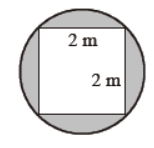(A) (2π – 4) m3
(B) (4π – 4) m3
(C) (4π – 2) m3
(D) (2π – 2) m3

Question. Two circular cylinders of equal volume have heights in the ratio of 1 “ 2. The ratio of their radii is
(A) 1 : 2
(B) 2 : 1
(C) 1: √2
(D) √2 :1

Question. A rectangle ground is 90 m long and 32 m broad. In the middle of the ground there is a circular tank of radius 14 meters. The cost of turfing the remaining portion at the rate of Rs. 2.50 per square meter is
(A) Rs. 6000
(B) Rs. 5660
(C) Rs. 5800
(D) Rs. 5750

Question. If the diagonal of a square is doubled, the area of the square becomes
(A) 2 times
(B) 3 times
(C) 4 times
(D) 6 times

Question. The area of the field if M is the mid point of LN is(A) 15200 sq. units
(B) 13300 sq. units
(C) 15000 sq. units
(D) 27800 sq. units

Question. The area of the trapezium ABCD as shown in the figure (in square units) is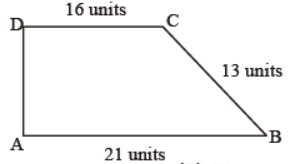(A) 222
(B) 111
(C) 224
(D) 116

Question. What is the length of AC? (All dimensions are in cm)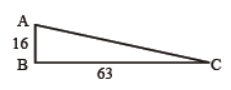(A) 95
(B) 75
(C) 85
(D) 65

Question. What is the volume of the rectangular solid shown below ?(A) 520 cm3
(B) 725 cm3
(C) 420 cm3
(D) 720 cm3

Question. The hypotenuse of a triangle is 29 cm and one of its sides is 20 cm, then the other side is
(A) 20 cm
(B) 21 cm
(C) 27 cm
(D) 25 cm

Question. A hoop is resting vertically at stair case as shown in the diagram. AB = 12 cm and BC = 8 cm. The radius of the hoop is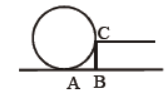(A) 13 cm
(B) 12 √2 cm
(C) 14 cm
(D) 13 √2 cm

Question. O is the centre of the regular pentagon. What part of the whole pentagon is the shaded region ?(A) 10 %
(B) 20 %
(C) 25 %
(D) 30 %

Question. The perimeter of the figure given below correct to one decimal place is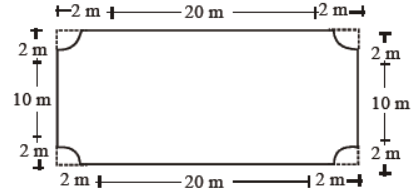(A) 56.0 m
(B) 56.6 m
(C) 57.2 m
(D) 57.9 m

Question. The ratio between the length and the perimeter of a rectangular plot is 1 : 3 and the ratio between the breadth and perimeter of that plot is 1 : 6. What is the ratio between the length and area of that plot ?
(A) 2 : 1
(B) 1 : 6
(C) 1 : 8

Question. A cylindrical tower is 5 m in diameter and 14 meter high. The cost of white washing its curved surface at 50 paisa per m2 is
(A) Rs. 90
(B) Rs. 97
(C) Rs. 110
(D) Rs. 95

Question. A square garden measuring 8 m on a side is surrounded by a 1 m wide path. What is the area of the path?(A) 8 square m
(B) 9 square m
(C) 28 square m
(D) 36 square m

Question. In the figure below, LMNO and GHJK are rectangles where GH = 1/2 LM and HJ = 1/2 MN. What fraction of the region is bounded by LMNO that is not shaded ?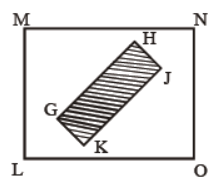(A) 1/4
(B) 1/3
(C) 1/2
(D) 3/4

Question. Each side of cube is increased by 50%. Then the surface area of the cube increase by
(A) 50 %
(B) 100 %
(C) 125 %
(D) 150 %

Question. In the figure below, RSTV is a square inscribed in a circle with centre O and radius r. The total area of shaded region is(A) r2(π – 2)
(B) 2r2(2 – π)
(C) π(r2 – 2)
(D) 8r2 – 8r

Question. The length of diagonal of a square whose area is 16900 m2 is
(A) 130 m
(B) 130 2 m
(C) 169 m
(D) 144 m

Question. The dimensions of a hall are 40 m, 25 m and 20 m. If each person requires 200 cubic meter, then the number of persons who can be accommodated in the hall are
(A) 120
(B) 150
(C) 140
(D) 100

Question. What is the ratio of the perimeter of the shaded region to the circumference of the circle ?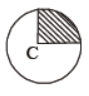(A) 3/4
(B) 4+π/4π
(C) 2π/4+π
(D) 4+π/2π

Question. The volume of a cube is 512 cm3. Its surface area is
(A) 64 cm2
(B) 256 cm2
(C) 384 cm2
(D) 512 cm2

Question. In rectangle ABCD, if BC/BE = CD/DF = √3 . If BE = 6 cm and AF = 2 cm, which of the following integers is nearest to the area of the rectangle ABCD in sq. cm. ?(A) 130
(B) 150
(C) 170
(D) 190

Question. In an n sides regular polygon the radius of the circum-circle is equal in length to the shortest diagonal, then n is
(A) 6
(B) 8
(C) 12
(D) Such a polygon does not exist

Question. The sum of sides of cube and rectangular parallelopiped are same. The ratio of volume of cube to that of parallelopied is
(A) 1
(B) Less than 1
(C) Greater than 1
(D) None of these

Question. The area of a rhombus is 120 cm2 and its altitude is 10 cm. The length of the rhombus is
(A) 24 cm
(B) 12 cm
(C) 8 cm
(D) 4 cm

Question. A quadrilateral whose one diagonal is 5.5 cm. If the length of altitudes from opposite vertices on the diagonal is 2.5 cm and 1.5 cm, then the area of quadrilateral is
(A) 22 cm2
(B) 11 cm2
(C) 55 cm2
(D) 33 cm2

Question. Vertical and horizontal cross sections of a right circular cylinder are always respectively
(A) rectangle, square
(B) rectangle, circle
(C) square, circle
(D) rectangle, ellipse

Question. The surface area of the cube given, is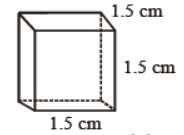(A) 3.375 cm2
(B) 13.5 cm2
(C) 6.75 cm2
(D) 9 cm2

Question. Bases of four equilateral triangles form a square. Inside the square four circle of radius 5 units are drawn as in the figure. The perimeter of the four cornered star is(A) 100
(B) 120
(C) 160
(D) 200

Question. The diameter of the circle in the picture is 10 cm. The perimeter of the region marked in thick line in cms is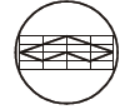(A) 12
(B) 16
(C) 20
(D) 24

Question. The diagonal of a isosceles trapezium is 16 cm long and marks an angle of 45°(A) 64 cm2
(B) 96 cm2
(C) 128 cm2
(D) 256 cm2

Question. The radius of a cylinder is doubled but its lateral surface area of unchanged. The its height must be
(A) Doubled
(B) Halved
(C) Trebled
(D) Constant

Question. The perimeter of a trapezium is 52 cm and its non-parallel sides are each equal to 10 cm and its altitude is 8 cm. Its area is
(A) 128 cm2
(B) 112 cm2
(C) 118 cm2
(D) 124 cm2

Question. Total area of quadrilateral ABCD is 20 cm2 and offsets on BD are 2 cm and 3 cm. The length of BD is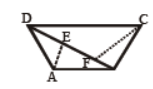(A) 5 cm
(B) 6 cm
(C) 8 cm
(D) 10 cm

Question. How many small cubes with edges of 10 cm can be just accommodated in a cubical box of 1 m edge?
(A) 10
(B) 100
(C) 1000
(D) 10000

Question. The largest possible square is inscribed in a circle of unit radius. The area of the square in square units is
(A) 2
(B) π
(C) 2 √2π
(D) 4 √2π

Question. In this scale drawing of a molding, each square represents 1 square inch. What is the area of the molding?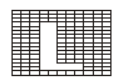(A) 24 sq. in
(B) 34 sq. in
(C) 35 sq. in
(D) 37 sq. in

Question. The given figure below shows a square inscribed in a larger square. What is the area of the smaller square ?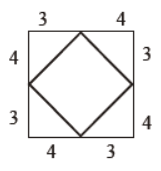(A) 9 square units
(B) 16 square units
(C) 25 square units
(D) 36 square units

#### Question: 1cm3 = ________

• a) 1000 cubic mm
• b) 10000m3
• c) 1000m3
• d) 10m3

• a) 1000
• b) 10,000
• c) 100,000
• d) 10,00,000

• a) 1
• b) 10
• c) 100
• d) 1000

• a) 9700
• b) 970
• c) 97
• d) 97000

• a) 6m
• b) 5m
• c) 7m

• a) 64cm3
• b) 24cm3
• c) 12cm3

#### Question: If the length of each edge of a cube is tripled, the change in it volume will be.

• a) 27 times of original
• b) 9 times of the original
• c) Both

• a) 96cm2
• b) 84cm2
• c) 32cm2
• d) 64cm2

• a) 1350cm2
• b) 1250cm2
• c) 1150cm2
• d) 1050cm2

• a) three
• b) four
• c) two

• a) 6 faces
• b) 12 faces
• c) 8 faces
• d) 4 faces

#### Question: A cuboid have

• a) 8 vertices
• b) 12 vertices
• c) 6 vertices

• a) 12 edges
• b) 12 edges
• c) 10 edges
• d) 6 edges

• a) 12cm
• b) 18cm
• c) 14cm
• d) 16cm

• a) Capacity
• b) height
• c) Area

#### Question: The most common unit of capacity in the metric system is

• a) litre
• b) cm3
• c) millilitre

• a) 1000000
• b) 100000
• c) 10000
• d) 1000

#### Question: A cubid measures 16cm × 10cm × 5cm. What happens to the volumes, when the dimensions of the cuboid are doubled?

• a) The volume is multiplied by 8
• b) The volume is multiplied by 2
• c) The volume reduces by one- half
• d) The volume is multiplied by 1/8

Answer: The volume is multiplied by 8

• a) 20cm
• b) 25cm
• c) 30cm
• d) 35cm

• a) 27kg
• b) 18kg
• c) 36kg

• a) 343
• b) 274
• c) 334

• a) 5cm
• b) 4cm
• c) 6cm

• a) 8 times
• b) 16 times
• c) 6 times

#### Question: A company is deciding on which box to be used to package their product. The first box measures 15cm by 8cm by 6.25cm. The second box measures 18cm by 6cm by 5.5cm. Which box requires more material to make?

• a) first box
• b) second box
• c) Both

 CBSE Class 9 Maths Number Systems MCQs
 CBSE Class 9 Maths Circles MCQs
 CBSE Class 9 Maths Constructions MCQs
 CBSE Class 9 Maths Herons Formula MCQs
 CBSE Class 9 Maths Surface Areas and Volumes MCQs
 CBSE Class 9 Maths Statistics MCQs
 CBSE Class 9 Maths Probability MCQs
 CBSE Class 9 Maths Polynomials MCQs
 CBSE Class 9 Maths Coordinate Geometry MCQs
 CBSE Class 9 Maths Linear Equations in Two Variables MCQs
 CBSE Class 9 Maths Introduction to Euclids Geometry MCQs
 CBSE Class 9 Maths Lines and Angles MCQs
 CBSE Class 9 Maths Triangles MCQs
 CBSE Class 9 Maths Quadrilaterals MCQs
 CBSE Class 9 Maths Areas of Parallelogram and Triangle MCQs
 CBSE Class 9 Mathematics MCQs CBSE Class 9 Quantitative Reasoning MCQs